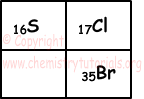## Strengths of Acids and Bases

Strengths of Acids and Bases

Strong Acids and Weak Acids:

Strength of acid is related to ionization of acids in water. Some of the acids can ionize 100 % in water solutions, we call them "strong acids". HCl, HNO3, HBr, HI, H2SO4 are examples of strong acids. Example given below show molar concentration of H+ ion in water solution of HCl and HNO3;

HCl(aq)  → H+(aq) + Cl-(aq)

0,1mol/L   0,1M       0,1M

Concentration of H+ ion is [H+]=0,1 M

HNO3(aq) → H+(aq) + NO3-(aq)

0,1mol/L      0,1M       0,1M

Concentration of H+ ion is [H+]=0,1 M

On the contrary, some of the acids can not ionize like strong acids. We call acids partially ionize in solutions "weak acid". CH3COOH, HF, H2CO3 are examples of weak acids. When weak acids dissolve in water;

CH3COOH (aq) ↔ H+(aq) CH3COO-(aq)

HF(aq) ↔ H+(aq) + F-(aq)

There are 1% ionization in 0,1 molar CH3COOH solution. Amount of CH3COOH in 1 L water is;

0,1.(1/100)=0,001 mol CH3COOH

Amounts of H+ ion and CH3COOH- ions are also 0,001 mol.

As a result; 0,1-0,001=0,099 mol CH3COOH is not ionized.

As we said before acid solutions conduct electric current. Electric current is directly proportional to ion concentration in the solution. Thus, we can say that solutions of strong acids conduct electricity better than solutions of weak acids.

Let X be any element;

• If electronegativity of X increases than strength of acid produced by X and H also increases.
• If energy between bonds of X and H increases, then strength of acid decreases.
• In periodic table, from top to bottom, in same group, H-X strength increases.

Example:

HI>HBr>HCl>HF

• In periodic table, from left to right strength of H-X increases.

Example:

HF>H2O>NH3>CH4

Strong and Weak Bases:

Bases ionize completely in solutions are called "strong bases". NaOH, KOH, Ba(OH)2 and bases including OH- ion are strong bases.

NaOH(aq) → Na+2(aq) + OH-(aq)

Ba(OH)2(aq) → Ba+2(aq) + 2OH-(aq)

Bases that ionize partially in solutions are called "weak bases". NH3 is an example of weak base.

NH3(aq) + H2O(l) ↔ NH4+(aq) OH-(aq)

Water solutions of bases also conduct electricity and it is directly proportional to ion concentration in solution. Thus, solutions of strong bases conduct electricity better than solutions of weak bases.

• In periodic table, from top to bottom in metal groups base strength of compounds increases.

Example:

LiOH<NaOH<KOH

• In periodic table from left to right, base strength of compounds decreases.

Example:

NaOH>Mg(OH)2>Al(OH)3

Example: Find relation between strength of acids of following elements with H shown in periodic table.Solution: Strength of H compounds increases as we go from left to right and top to bottom in periodic table. Thus, for given elements;

HCl>H2S and HBr>HCl

HBr>HCl>H2S

Acids and Bases Exams and  Problem Solutions

Related